For those wishing to follow along with the R-based demo in class, click here for the companion R-script for this lecture.

If you haven’t already done this, please sign up (on our shared Google Sheets) for a time slot (30 mins) to discuss your group project proposals with me on Tues Oct 1. The rest of lab time is yours to either work on the group projects or get started with lab 3 (if I finish revising it in time!)

# Optimization

We can’t maximize a likelihood function without an optimization algorithm.

We can’t optimize a sampling or monitoring regime, as in the power analysis problem, without an optimization algorithm.

Clearly, we need optimization algorithms!! In addition, they provide an excellent example of how computers (often via brute force algorithms) can supersede pure mathematics for performing statistical analysis.

You may not have built your own optimization algorithm before, but you’ve probably taken advantage of optimization algorithms that are operating behind the scenes. For example, if you have performed a glmm or a non-linear regression in R, you have exploited numerical optimization routines!

We will discuss optimization in the context of maximum likelihood estimation, and a couple lectures from now we’ll discuss optimization in a Bayesian context. Let’s start with the most simple of all optimization algorithms – brute force!

Note: you won’t need to build your own optimization routines for this class- the code in this lecture is for demonstration purposes only!

## Brute Force!

Just like we did for the two-dimensional likelihood surface, we could evaluate the likelihood at tiny intervals across a broad range of parameter space. Then we can just identify the parameter set associated with the maximum likelihood across all evaluated parameter sets.

### Positives

• Simple!! (conceptually very straightforward)
• Identify false peaks! (guaranteed to find the MLE!)
• Undeterred by discontinuities in the likelihood surface

### Negatives

• Speed: even slower and less efficient than a typical ecologist is willing to accept!
• Resolution: we may specify the wrong interval size. Even so, we can only get the answer to within plus or minus the interval size.

### Example dataset: Myxomatosis titer in rabbits

Let’s use Bolker’s myxomatosis example dataset (an example we’ll return to frequently!) to illustrate:

####################
# Explore Bolker's myxomatosis example

library(emdbook)    # this is the package provided to support the textbook!

MyxDat <- MyxoTiter_sum         # load Bolker's example data
head(Myx)
##   grade day titer
## 1     1   2 5.207
## 2     1   2 5.734
## 3     1   2 6.613
## 4     1   3 5.997
## 5     1   3 6.612
## 6     1   3 6.810

For this example, we are modeling the distribution of measured titers (virus loads) for Australian rabbits. Bolker chose to use a Gamma distribution. Here is the empirical distribution:

hist(Myx$titer,freq=FALSE) # distribution of virus loads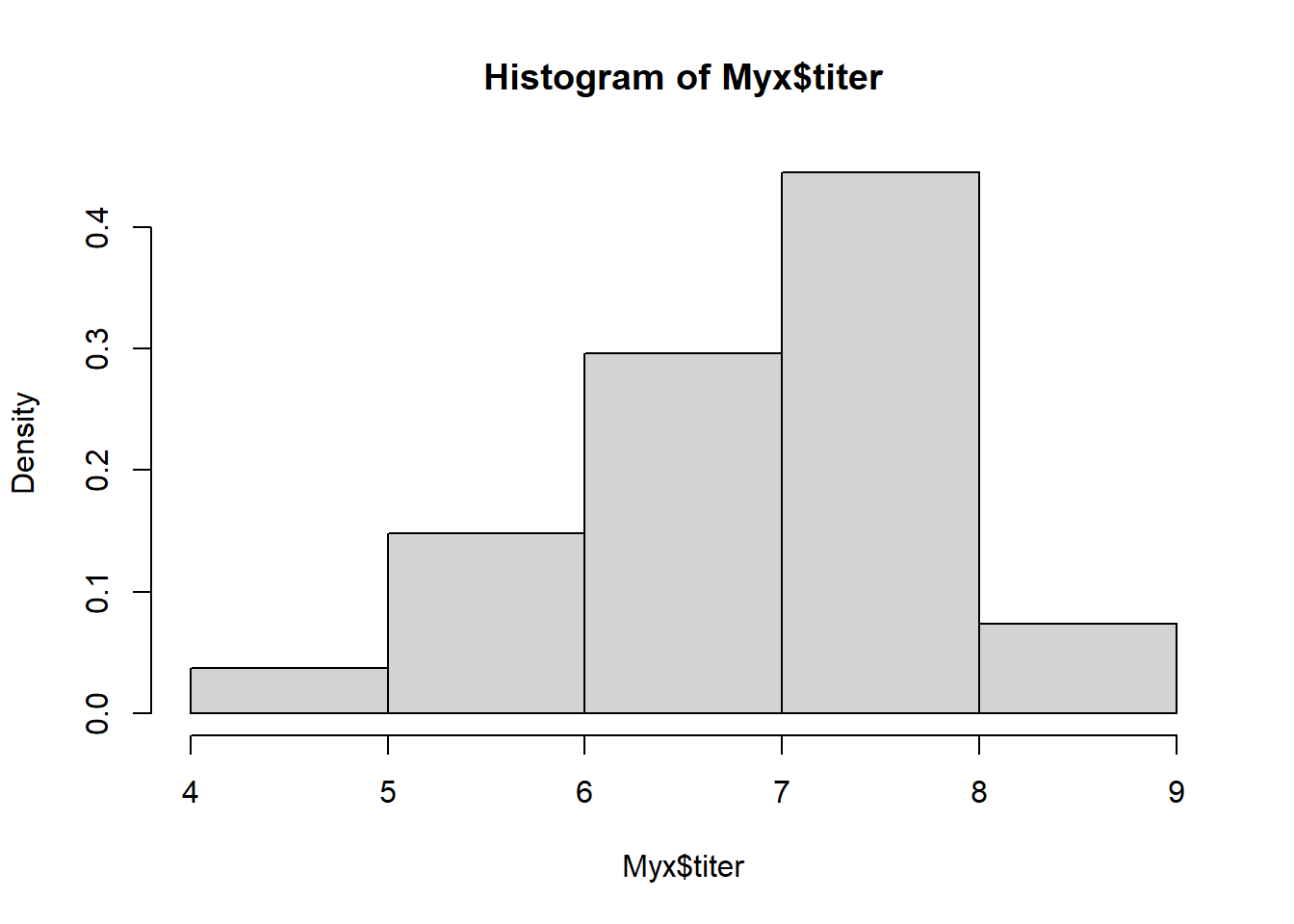We need to estimate the gamma ‘rate’ and ‘shape’ parameters that best fit this empirical distribution. Here is one example of a Gamma fit to this distribution: ########### # Overlay a gamma distribution on the histogram hist(Myx$titer,freq=FALSE)     # note the "freq=FALSE", which displays densities of observations, and therefore makes histograms comparable with probability density functions
curve(dgamma(x,shape=40,scale=0.15),add=T,col="red")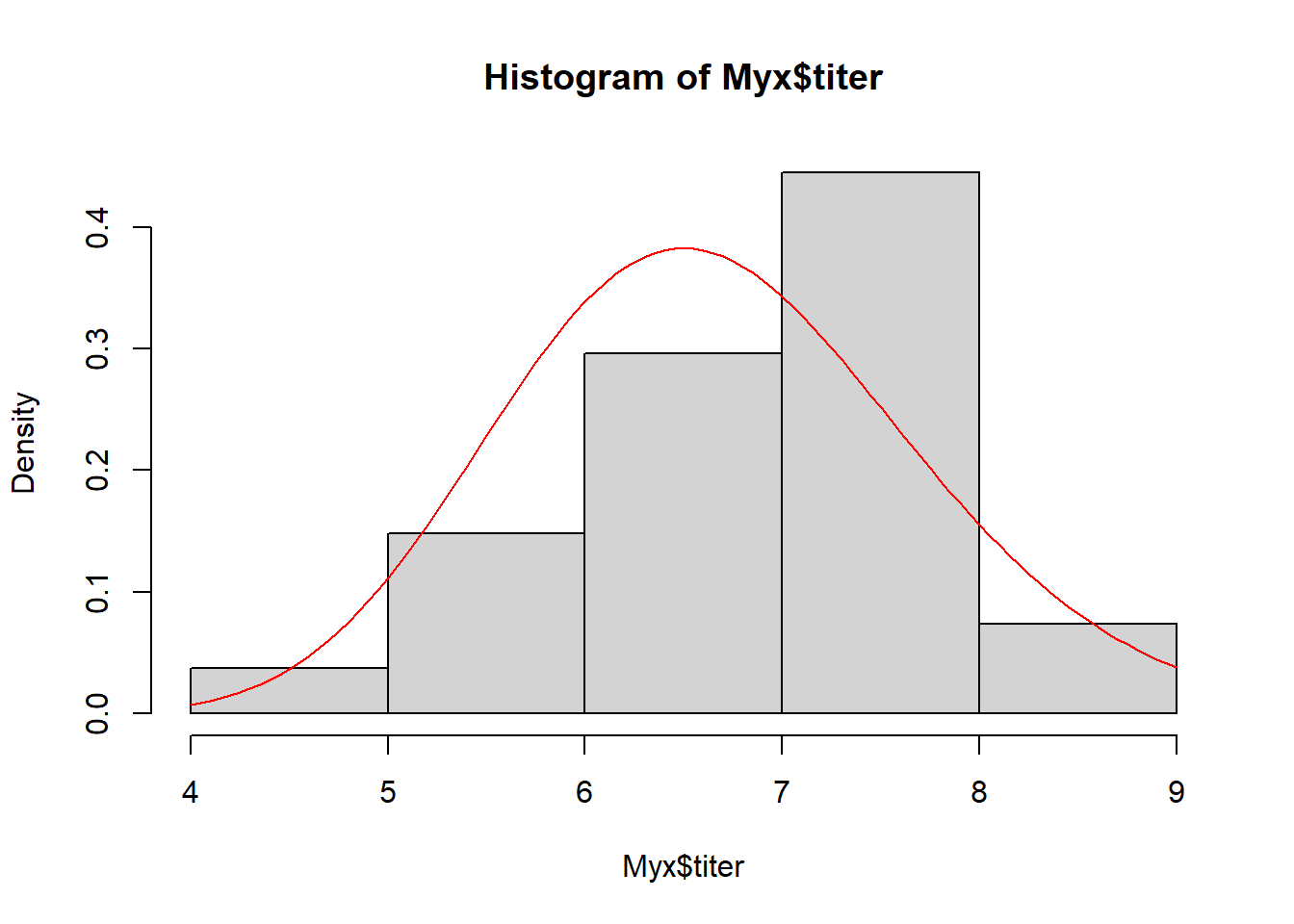This is clearly not a great fit, but perhaps this would be an okay starting point (optimization algorithms don’t really require perfect starting points, just need to be in the ballpark)…

Let’s build a likelihood function for this problem!

################
# Build likelihood function

GammaLikelihoodFunction <- function(params){           # only one argument (params)- the data are hard-coded here (this is often the case with simple likelihood functions)
sum(dgamma(Myx$titer,shape=params['shape'],scale=params['scale'],log=T)) # use params and data to compute likelihood } params <- c(40,0.15) names(params) <- c("shape","scale") params ## shape scale ## 40.00 0.15 GammaLikelihoodFunction(params) # test the function! ##  -49.58983 Now let’s optimize using ‘optim()’ like we did before, to find the MLE! NOTE: “optim()” will throw some warnings here because it will try to find the data likelihood for certain impossible parameter combinations! ############ # USE R's 'OPTIM()' FUNCTION ############ ############ # Optimize using R's built-in "optim()" function: find the maximum likelihood estimate ctrl <- list(fnscale=-1) # maximize rather than minimize!! MLE <- optim(fn=GammaLikelihoodFunction,par=params,control=ctrl,method="BFGS") # stop the warnings! ## Warning in dgamma(Myx$titer, shape = params["shape"], scale =
## params["scale"], : NaNs produced

## Warning in dgamma(Myx$titer, shape = params["shape"], scale = ## params["scale"], : NaNs produced ## Warning in dgamma(Myx$titer, shape = params["shape"], scale =
## params["scale"], : NaNs produced

## Warning in dgamma(Myx$titer, shape = params["shape"], scale = ## params["scale"], : NaNs produced ## Warning in dgamma(Myx$titer, shape = params["shape"], scale =
## params["scale"], : NaNs produced

## Warning in dgamma(Myx$titer, shape = params["shape"], scale = ## params["scale"], : NaNs produced ## Warning in dgamma(Myx$titer, shape = params["shape"], scale =
## params["scale"], : NaNs produced

## Warning in dgamma(Myx$titer, shape = params["shape"], scale = ## params["scale"], : NaNs produced ## Warning in dgamma(Myx$titer, shape = params["shape"], scale =
## params["scale"], : NaNs produced

## Warning in dgamma(Myx$titer, shape = params["shape"], scale = ## params["scale"], : NaNs produced ## Warning in dgamma(Myx$titer, shape = params["shape"], scale =
## params["scale"], : NaNs produced

## Warning in dgamma(Myx$titer, shape = params["shape"], scale = ## params["scale"], : NaNs produced ## Warning in dgamma(Myx$titer, shape = params["shape"], scale =
## params["scale"], : NaNs produced

## Warning in dgamma(Myx$titer, shape = params["shape"], scale = ## params["scale"], : NaNs produced ## Warning in dgamma(Myx$titer, shape = params["shape"], scale =
## params["scale"], : NaNs produced

## Warning in dgamma(Myx$titer, shape = params["shape"], scale = ## params["scale"], : NaNs produced ## Warning in dgamma(Myx$titer, shape = params["shape"], scale =
## params["scale"], : NaNs produced

## Warning in dgamma(Myx$titer, shape = params["shape"], scale = ## params["scale"], : NaNs produced ## Warning in dgamma(Myx$titer, shape = params["shape"], scale =
## params["scale"], : NaNs produced

## Warning in dgamma(Myx$titer, shape = params["shape"], scale = ## params["scale"], : NaNs produced ## Warning in dgamma(Myx$titer, shape = params["shape"], scale =
## params["scale"], : NaNs produced

## Warning in dgamma(Myx$titer, shape = params["shape"], scale = ## params["scale"], : NaNs produced ## Warning in dgamma(Myx$titer, shape = params["shape"], scale =
## params["scale"], : NaNs produced

## Warning in dgamma(Myx$titer, shape = params["shape"], scale = ## params["scale"], : NaNs produced ## Warning in dgamma(Myx$titer, shape = params["shape"], scale =
## params["scale"], : NaNs produced

## Warning in dgamma(Myx$titer, shape = params["shape"], scale = ## params["scale"], : NaNs produced ## Warning in dgamma(Myx$titer, shape = params["shape"], scale =
## params["scale"], : NaNs produced
MLE$par ## shape scale ## 49.3666607 0.1402629 We can ignore the warnings! Let’s visualize the fit of the MLE in this case… ############## # visualize the fit hist(Myx$titer,freq=FALSE)
curve(dgamma(x,shape=MLE$par["shape"],scale=MLE$par["scale"]),add=T,col="red")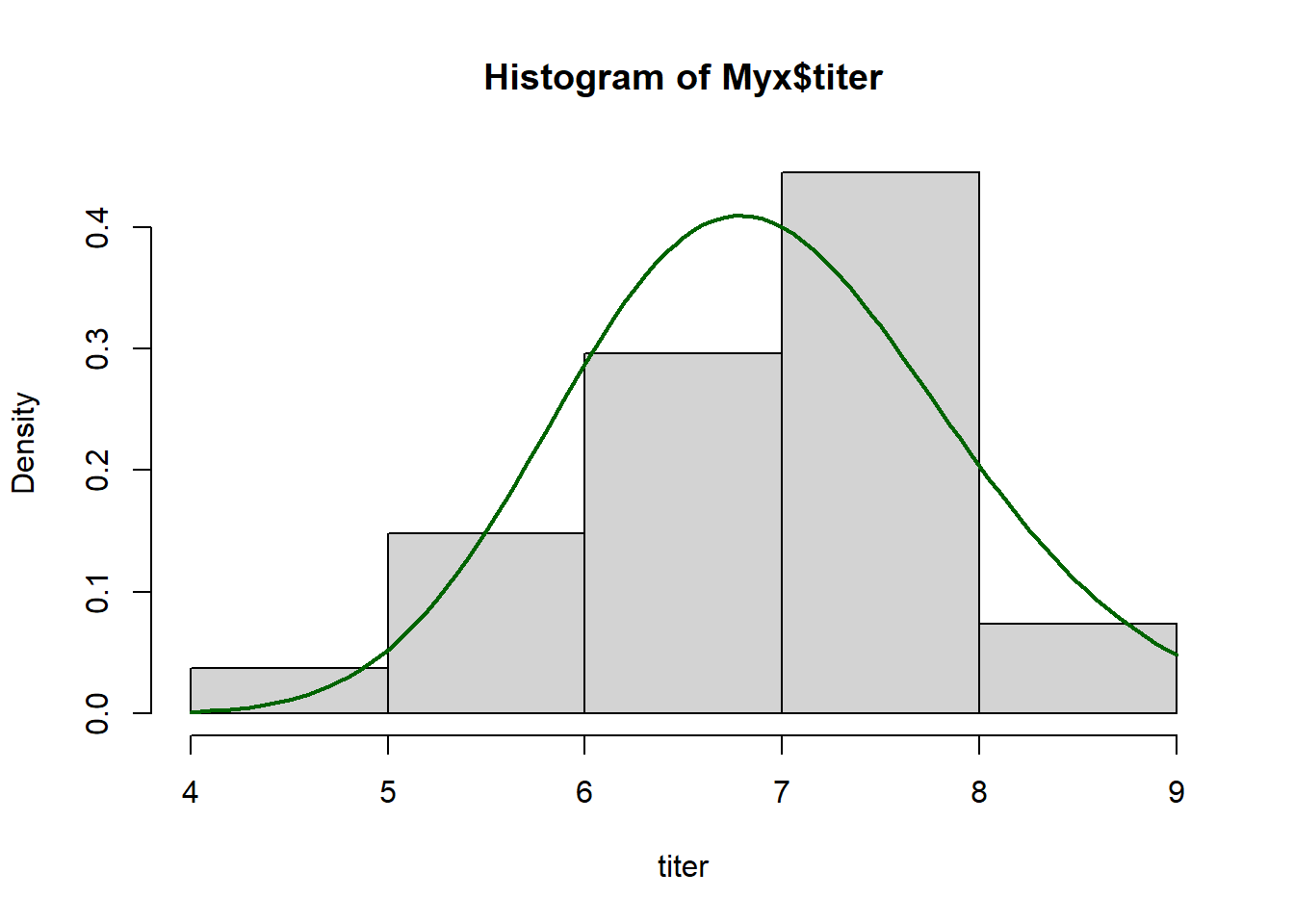Looks pretty good…

But as dangerous ecological statisticians we aren’t satisfied with using a “black box” like the “optim()” function, we need to understand what is going on behind the scenes. Let’s write our own optimizer!

We start with the conceptually simple, often computationally impossible, brute force method…

######################
# BRUTE FORCE ALTERNATIVE
######################

##############
# define 2-D parameter space!
##############

shapevec <- seq(10,100,by=0.1)
scalevec <- seq(0.01,0.3,by=0.001)

##############
# define the likelihood surface across this grid within parameter space
##############

surface2D <- matrix(nrow=length(shapevec),ncol=length(scalevec))   # initialize storage variable

newparams <- params
for(i in 1:length(shapevec)){
newparams['shape'] <- shapevec[i]
for(j in 1:length(scalevec)){
newparams['scale'] <- scalevec[j]
surface2D[i,j] <- GammaLikelihoodFunction(newparams)   # compute likelihood for every point in 2-d parameter space
}
}

############
# Visualize the likelihood surface
############

image(x=shapevec,y=scalevec,z=surface2D,zlim=c(-1000,-30),col=topo.colors(12))
contour(x=shapevec,y=scalevec,z=surface2D,levels=c(-30,-40,-80,-500),add=T)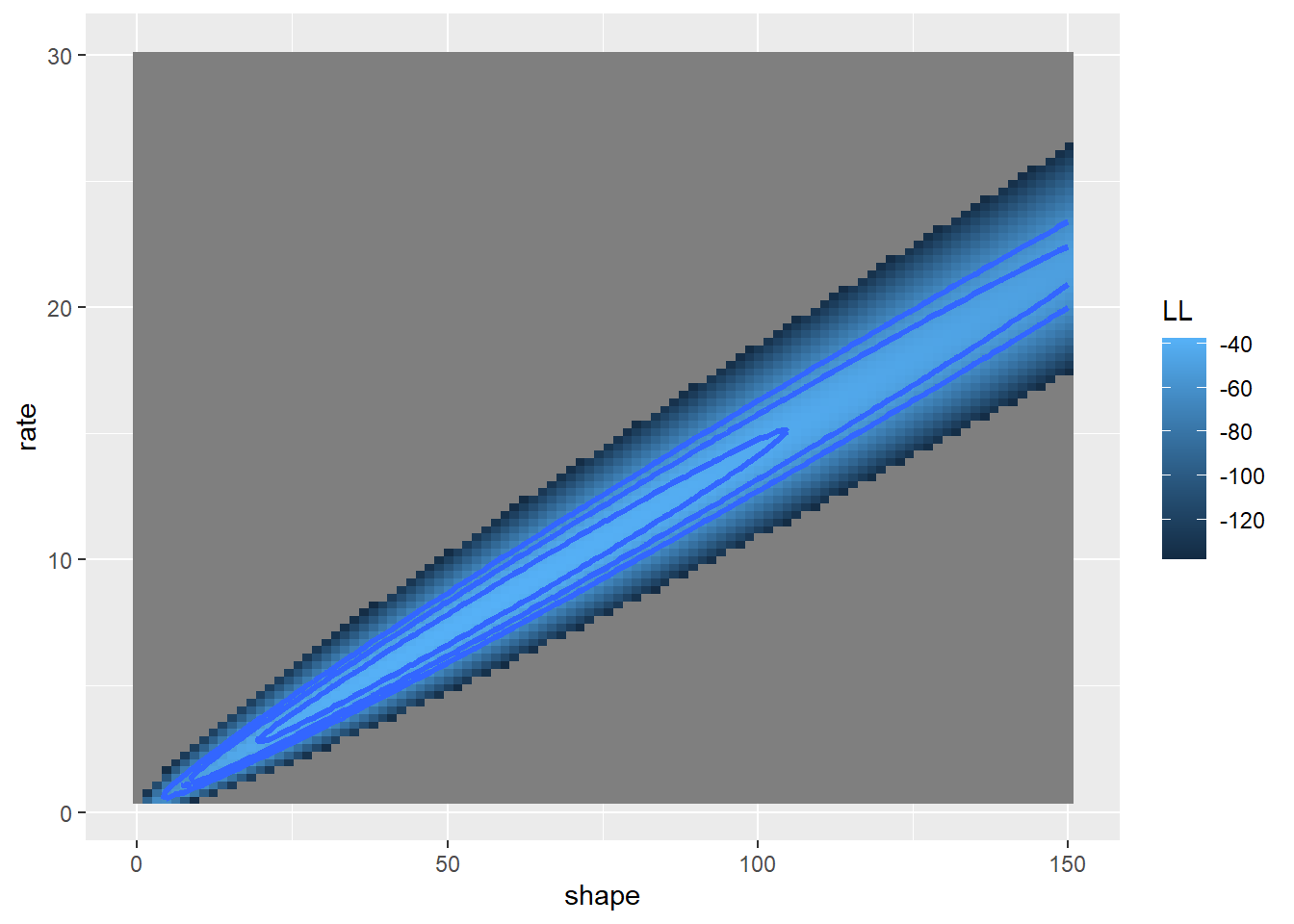Now what is the maximum likelihood estimate?

############
# Find the MLE
############

ndx <- which(surface2D==max(surface2D),arr.ind=T)  # index of the max likelihood grid cell
shapevec[ndx[,1]]     
##  49.8
scalevec[ndx[,2]]
##  0.139
MLE$par # compare with the answer from "optim()" ## shape scale ## 49.3666607 0.1402629 ## Derivative based methods! If we assume that the likelihood surface is smooth (differentiable) and has only one minimum, we can develop very efficient optimization algorithms. In general, derivative-based methods look for the point in parameter space where the derivative of the likelihood function is zero. That is, at the peak! Let’s imagine we are interested in determining the shape parameter, given a known scale parameter for a gamma distribution. To use derivative based methods, let’s first build a function that estimates the slope of the function at any arbtrary point in parameter space: ################### # Derivative-based optimization methods ################### ###### # function for estimating the slope of the likelihood surface at any point in parameter space.... ## NOTE: even here I'm using a coarse, brute force method for estimating the first and second derivative of the likelihood function params <- MLE$par
SlopeFunc <- function(shape_guess,tiny=0.001){
params['shape'] <- shape_guess
high <- GammaLikelihoodFunction(params+c(tiny,0))
low <- GammaLikelihoodFunction(params-c(tiny,0))
slope <- (high-low)/(tiny*2)
return(slope)
}

SlopeFunc(shape_guess=30)    #try it!
##  13.62666

Now let’s visualize this!

#########
# Visualize the slope of the likelihood function at different points in parameter space

shapevec <- seq(10,100,by=0.1)

##############
# define the likelihood surface
##############

surface1D <- numeric(length(shapevec))   # initialize storage variable

newparams <- params
for(i in 1:length(shapevec)){
newparams['shape'] <- shapevec[i]
surface1D[i] <- GammaLikelihoodFunction(newparams)
}

plot(surface1D~shapevec,type="l")
point <- GammaLikelihoodFunction(c(shape=30,MLE$par['scale'])) slope <- SlopeFunc(shape_guess=30) lines(c(20,40),c(point-slope*10,point+slope*10),col="red")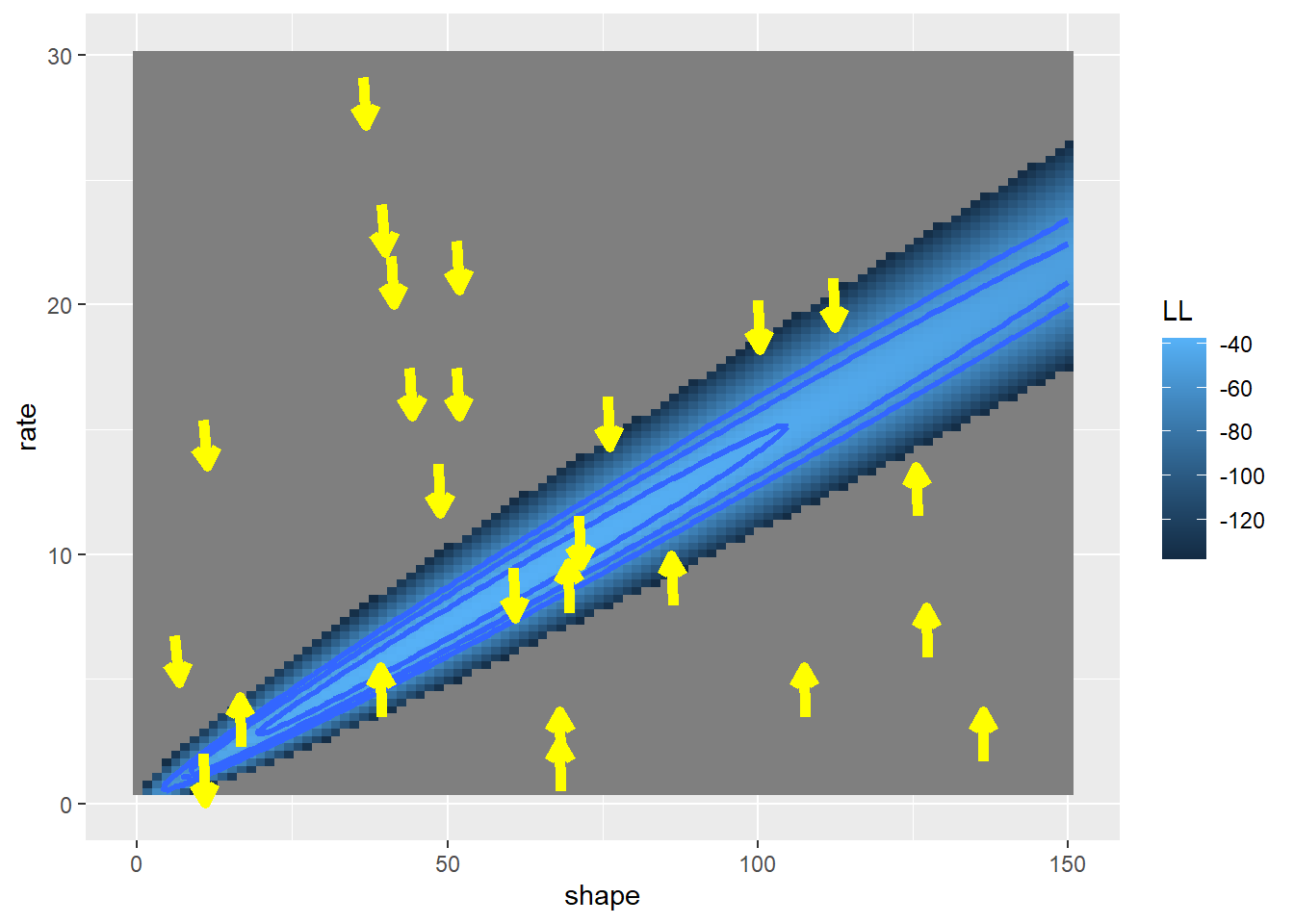We also need a function to compute the second derivative, or the curvature… ######## # function for estimating the curvature of the likelihood function at any point in parameter space params <- MLE$par
CurvatureFunc <- function(shape_guess,tiny=0.001){
params['shape'] <- shape_guess
high <- SlopeFunc(shape_guess+tiny)
low <- SlopeFunc(shape_guess-tiny)
curvature <- (high-low)/(tiny*2)   # how much the slope is changing in this region of the function
return(curvature)
}

CurvatureFunc(shape_guess=30)   # try it!
##  -0.9151666

Okay, now we can implement a derivative-based optimization algorithm!

Essentially, we are trying to find the point where the derivative of the likelihood function is zero (the root of the function!).

The simplest derivative-based optimization algorithm is the Newton-Raphson algorithm. Here is the pseudocode:

• pick a guess for a parameter value
• compute the derivative of the likelihood function for that guess
• compute the slope of the derivative (curvature) of the likelihood function for that guess
• Extrapolate linearly to try to find the root (where the derivative of the likelihood function should be zero if the slope of the likelihood function were linear)
• repeat until the derivative of the likelihood function is close enough to zero (within a specified tolerance)

Let’s first visualize the shape of the first derivative of the likelihood function

######
# First- visualize the gradient of the likelihood function

firstderiv <- numeric(length(shapevec))   # initialize storage variable
for(i in 1:length(shapevec)){
firstderiv[i] <- SlopeFunc(shapevec[i])
}

plot(firstderiv~shapevec,type="l")
abline(h=0,col="red")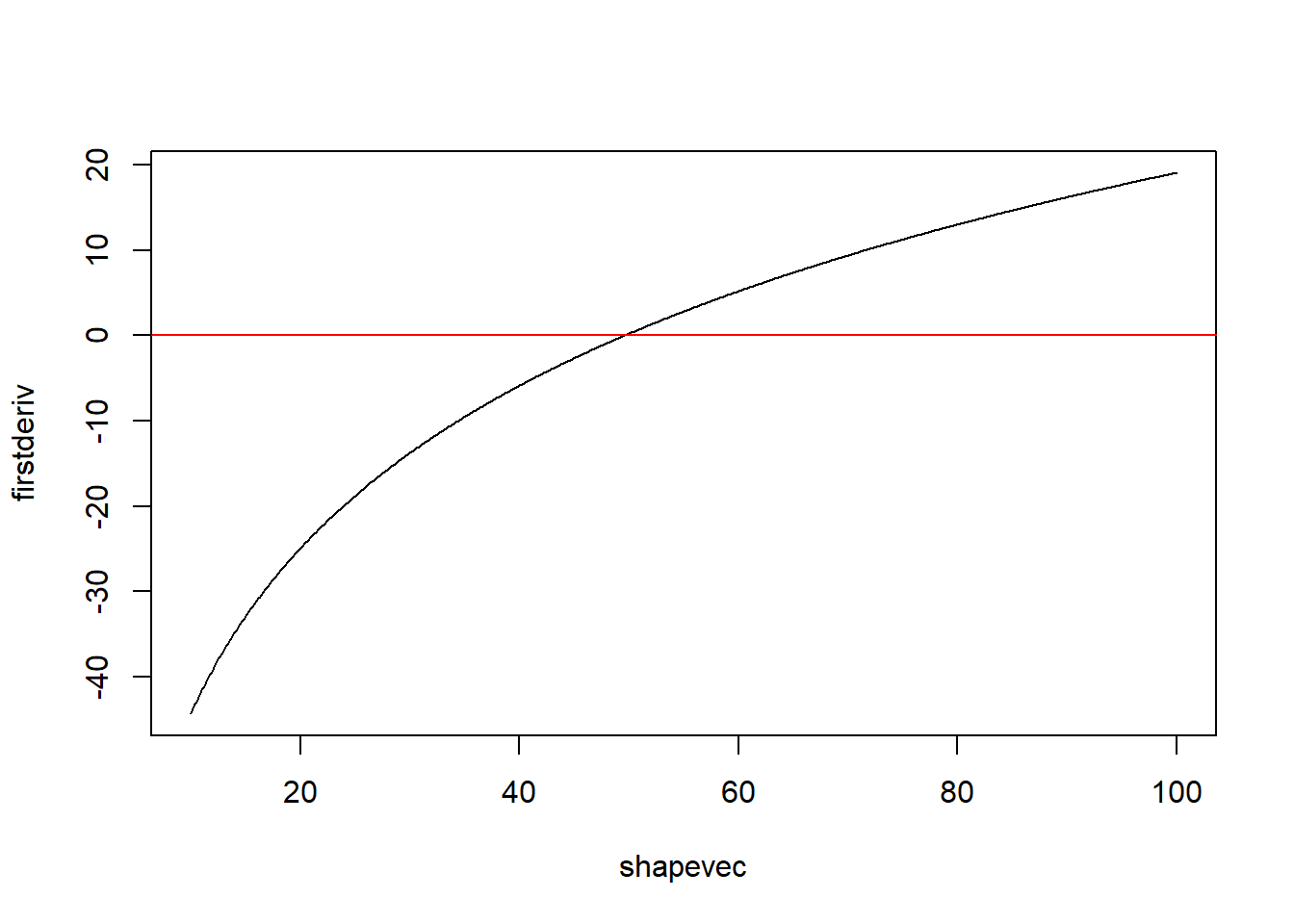Let’s use the Newton method to find the root of the likelihood function. First we pick a starting value. Say we pick 80.

First compute the derivatives:

#########
# Now we can perform a simple, derivative-based optimization!

### Pick "80" as the starting value

firstderiv <- SlopeFunc(80)           # evaluate the first and second derivatives
secondderiv <- CurvatureFunc(80)
firstderiv
##  -13.13913
secondderiv
##  -0.3396182

Now let’s use this linear function to extrapolate to where the first derivative is equal to zero:

#########
# Use this info to estimate the root

oldguess <- 80
newguess <- oldguess - firstderiv/secondderiv   # estimate the root (where first deriv is zero)
newguess
##  41.31206

Our new guess is that the shape parameter is 41.31. Let’s do it again!

##########
# Repeat this process

oldguess <- 41.31
newguess <- oldguess - SlopeFunc(oldguess)/CurvatureFunc(oldguess)
newguess
##  48.66339

Okay, we’re already getting close to our MLE of around 49.36. Let’s do it again:

#######
# again...

oldguess<-newguess
newguess <- oldguess - SlopeFunc(oldguess)/CurvatureFunc(oldguess)
newguess
##  49.36237

And again!

#######
# again...

oldguess<-newguess
newguess <- oldguess - SlopeFunc(oldguess)/CurvatureFunc(oldguess)
newguess
##  49.36746

And again!!!

#######
# again...

oldguess<-newguess
newguess <- oldguess - SlopeFunc(oldguess)/CurvatureFunc(oldguess)
newguess
##  49.36746

Wow, in just a few steps we already basically found the true root. Let’s find the root for real, using an algorithm…

##########
# Implement the Newton Method as a function!

NewtonMethod <- function(firstguess,tolerance=0.0000001){
deriv <- SlopeFunc(firstguess)
oldguess <- firstguess
counter <- 0
while(abs(deriv)>tolerance){
deriv <- SlopeFunc(oldguess)
newguess <- oldguess - deriv/CurvatureFunc(oldguess)
oldguess<-newguess
counter=counter+1
}
mle <- list()
mle$estimate <- newguess mle$likelihood <- GammaLikelihoodFunction(c(shape=newguess,MLE$par['scale'])) mle$iterations <- counter
return(mle)
}

newMLE <- NewtonMethod(firstguess=80)
newMLE
## $estimate ##  49.36746 ## ##$likelihood
##  -37.6673
##
## $iterations ##  6 In just 6 steps we successfully identified the maximum likelihood estimate to within 0.0000001 of the true value! How many computations did we have to perform to use the brute force method? Hopefully this illustrates the power of optimization algorithms!! Note that this method and other derivative-based methods can work in multiple dimensions! The only constraint here is that the likelihood function is differentiable (smooth) ## Derivative-free optimization methods Derivative-free methods make no assumption about smoothness. In some ways, they represent a middle ground between the brute force method and the elegant but finnicky derivative-based methods- walking a delicate balance between simplicity and generality. ## Derivative-free method 1: simplex method This is the default opimization method for “optim()”! That means that R used this method for optimizing the fuel economy example from the previous lecture! #### Definition: Simplex A simplex is the multi-dimensional analog of the triangle. In two dimensions, the triangle is the simplest shape possible. It has just one more vertex than there are dimensions! In n dimensions, a simplex is defined by n+1 vertices. #### Pseudocode for Nelder-Mead simplex algorithm Set up an initial simplex in parameter space (often based on a user’s initial guess). NOTE: when you use the Nelder-Mead algorithm in “optim()” you only specify one initial value for each free parameter. “optim()”’s internal algorithm turns that initial guess into a simplex prior to starting the Nelder-Mead algorithm. Continue the following steps until your answer is good enough: • Start by identifying the worst vertex (the one with the lowest likelihood) • Take the worst vertex and reflect it across the center of the shape represented by the other vertices. • If the likelihood is higher for the reflected point, but not the highest of all vertices, then accept the new vertex. • If the likelihood is highest for the reflected point, increase the length of the jump! If it’s better, accept the new point. • If this jump was bad (lower likelihood) then try a point that’s only a smaller step away from the initial try. If it’s better, accept the new point. If not, accept the original reflected point. • If all reflections were worse than any other vertex, then contract the simplex around the current highest-likelihood vertex. Q: What does the simplex look like for a one-dimensional optimization problem? Q: Is this method likely to be good at avoiding false peaks in the likelihood surface? ### Example: Simplex method Step 1: Set up an initial simplex in parameter space ############# # SIMPLEX OPTIMIZATION METHOD! ############# ######## # set up an "initial" simplex firstguess <- c(shape=70,scale=0.22) # "user" first guess simplex <- list() # set up the initial simplex based on the first guess... simplex[['vertex1']] <- firstguess + c(3,0.04) simplex[['vertex2']] <- firstguess + c(-3,-0.04) simplex[['vertex3']] <- firstguess + c(3,-0.04) simplex ##$vertex1
## shape scale
## 73.00  0.26
##
## $vertex2 ## shape scale ## 67.00 0.18 ## ##$vertex3
## shape scale
## 73.00  0.18

Let’s plot the simplex…

    ## first let's make a function to plot the simplex on a 2-D likelihood surface...

temp <- as.data.frame(simplex)    # easier to work with data frame here
points(x=temp[1,c(1,2,3,1)], y=temp[2,c(1,2,3,1)],type="b",lwd=2,col=col)
}

image(x=shapevec,y=scalevec,z=surface2D,zlim=c(-1000,-30),col=topo.colors(12))
addSimplex(simplex)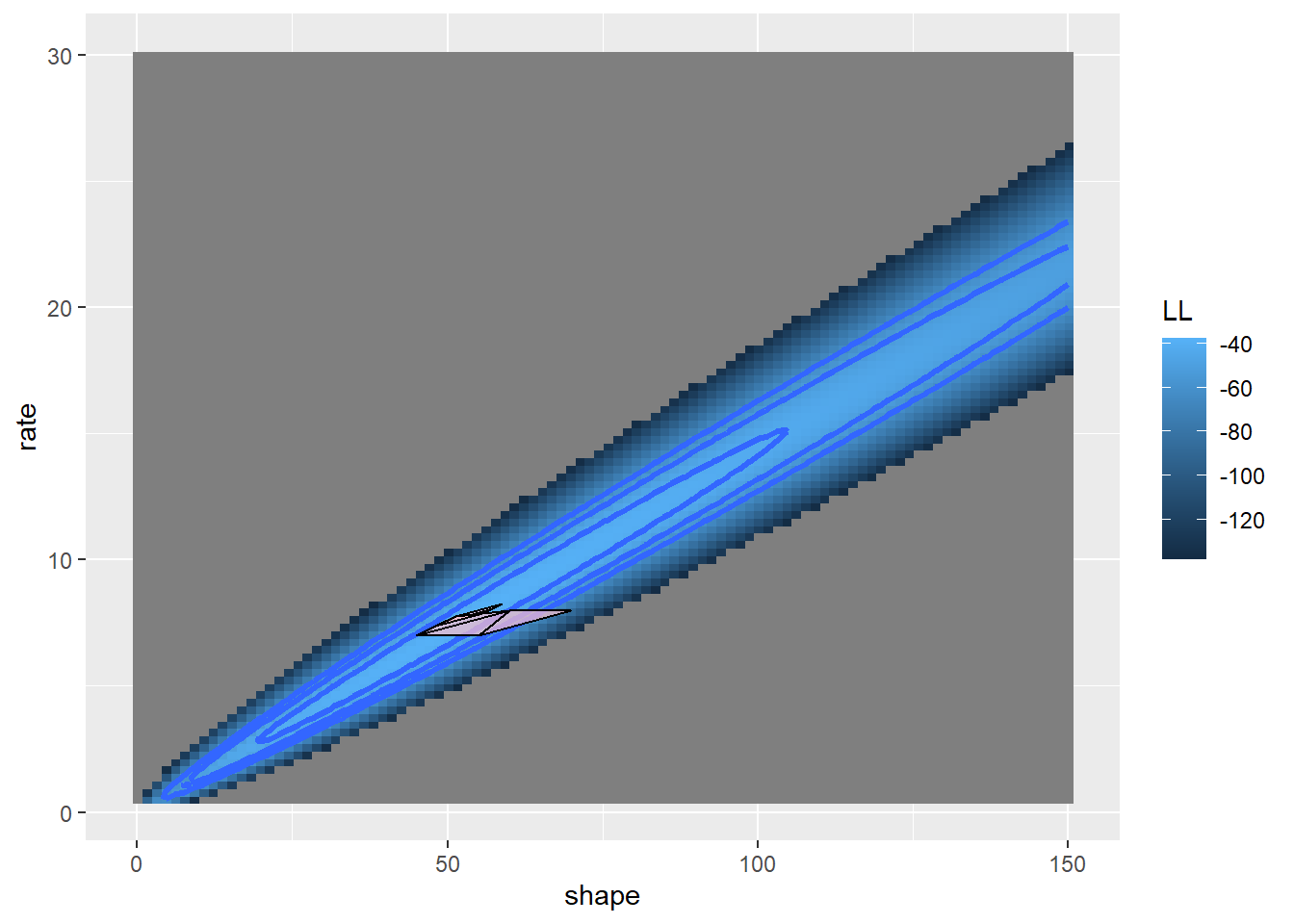Now let’s evaluate the log likelihood at each vertex

########
# Evaluate log-likelihood at each vertex of the simplex

SimplexLik <- function(simplex){
newvec <- unlist(lapply(simplex,GammaLikelihoodFunction))   # note use of apply instead of for loop...
return(newvec)
}

SimplexLik(simplex)
##   vertex1   vertex2   vertex3
## -774.3825 -271.7534 -369.1696

Now let’s develop a function (or functions as the case may be) to implement our first move through parameter space, according to the rules defined above…

#####
# Helper Functions
#####

## this function reflects the worst vertex across the remaining vector
ReflectIt <- function(oldsimplex,WorstVertex){

## re-arrange simplex- worst must be first
worstndx <- which(names(oldsimplex)==WorstVertex)
otherndx <- c(1:3)[-worstndx]
newndx <- c(worstndx,otherndx)

## translate so that vertex 1 is the origin (0,0)
oldsimplex <- oldsimplex[newndx]
translate <- oldsimplex[]
newsimplex <- list(oldsimplex[]-translate,oldsimplex[]-translate,oldsimplex[]-translate)

reflected <- c(newsimplex[]["shape"]+newsimplex[]["shape"],newsimplex[]["scale"]+newsimplex[]["scale"])
names(reflected) <- c("shape","scale")

## translate back to the likelihood surface
newsimplex[] <- reflected
newsimplex <- list(newsimplex[]+translate,newsimplex[]+translate,newsimplex[]+translate)
## return the new simplex
names(newsimplex) <- names(oldsimplex)

## generate some alternative jumps (or "oozes"!)...
oldpoint <- oldsimplex[]
newpoint <- newsimplex[]

newpoint2 <- newpoint-oldpoint
double <- newpoint2 * 3
half <- newpoint2 * 0.24

alternates <- list()
alternates$reflected <- newsimplex alternates$double <- newsimplex
alternates$half <- newsimplex alternates$double[] <- double + oldpoint
alternates$half[] <- half + oldpoint return(alternates) } ShrinkIt <- function(oldsimplex,BestVertex){ newsimplex <- oldsimplex ## indices... bestndx <- which(names(oldsimplex)==BestVertex) otherndx <- c(1:3)[-bestndx] translate <- oldsimplex[[bestndx]] i=2 for(i in otherndx){ newvector <- oldsimplex[[i]]-translate shrinkvector <- newvector * 0.5 newsimplex[[i]] <- shrinkvector + translate } return(newsimplex) } MoveTheSimplex <- function(oldsimplex){ # (incomplete) nelder-mead algorithm newsimplex <- oldsimplex # # Start by identifying the *worst* vertex (the one with the lowest likelihood) VertexLik <- SimplexLik(newsimplex) WorstLik <- min(VertexLik) BestLik <- max(VertexLik) WorstVertex <- names(VertexLik[which.min(VertexLik)]) # identify vertex with lowest likelihood candidates <- ReflectIt(oldsimplex=newsimplex,WorstVertex) # reflect across the remaining edge CandidateLik <- sapply(candidates,SimplexLik) # re-evaluate likelihood at the vertices... CandidateLik <- apply(CandidateLik,c(1,2), function(t) ifelse(is.nan(t),-99999,t)) bestCandidate <- names(which.max(CandidateLik[WorstVertex,])) bestCandidateLik <- CandidateLik[WorstVertex,bestCandidate] if(CandidateLik[WorstVertex,"reflected"]>=WorstLik){ if(CandidateLik[WorstVertex,"reflected"]>BestLik){ if(CandidateLik[WorstVertex,"double"]>CandidateLik[WorstVertex,"reflected"]){ newsimplex <- candidates[["double"]] # expansion }else{ newsimplex <- candidates[["reflected"]] } }else if(CandidateLik[WorstVertex,"half"]>CandidateLik[WorstVertex,"reflected"]){ # contraction newsimplex <- candidates[["half"]] }else{ newsimplex <- candidates[["reflected"]] } }else{ BestVertex <- names(VertexLik[which.max(VertexLik)]) newsimplex <- ShrinkIt(oldsimplex,BestVertex) } return(newsimplex) } # image(x=shapevec,y=scalevec,z=surface2D,zlim=c(-1000,-30),col=topo.colors(12)) # contour(x=shapevec,y=scalevec,z=surface2D,levels=c(-30,-40,-80,-500),add=T) # addSimplex(oldsimplex,col="red") # addSimplex(candidates$reflected,col="green")
# addSimplex(candidates$half,col="green") ########### # Visualize the simplex oldsimplex <- simplex newsimplex <- MoveTheSimplex(oldsimplex) ## Warning in dgamma(Myx$titer, shape = params["shape"], scale =
## params["scale"], : NaNs produced
image(x=shapevec,y=scalevec,z=surface2D,zlim=c(-1000,-30),col=topo.colors(12))
addSimplex(newsimplex)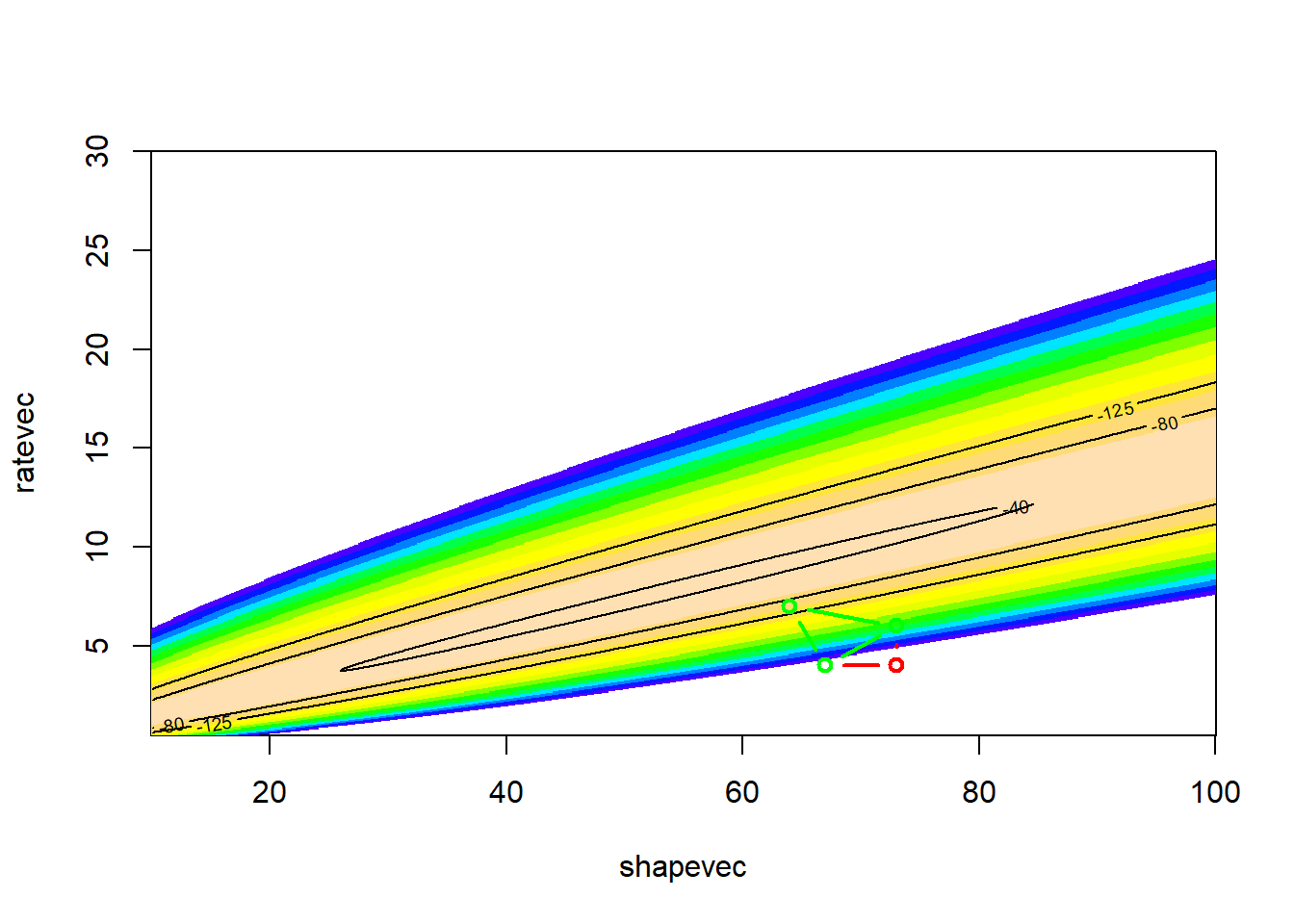Let’s try another few moves

############
# Make another move

oldsimplex <- newsimplex
newsimplex <- MoveTheSimplex(oldsimplex)
## Warning in dgamma(Myx$titer, shape = params["shape"], scale = ## params["scale"], : NaNs produced image(x=shapevec,y=scalevec,z=surface2D,zlim=c(-1000,-30),col=topo.colors(12)) contour(x=shapevec,y=scalevec,z=surface2D,levels=c(-30,-40,-80,-500),add=T) addSimplex(oldsimplex,col="red") addSimplex(newsimplex)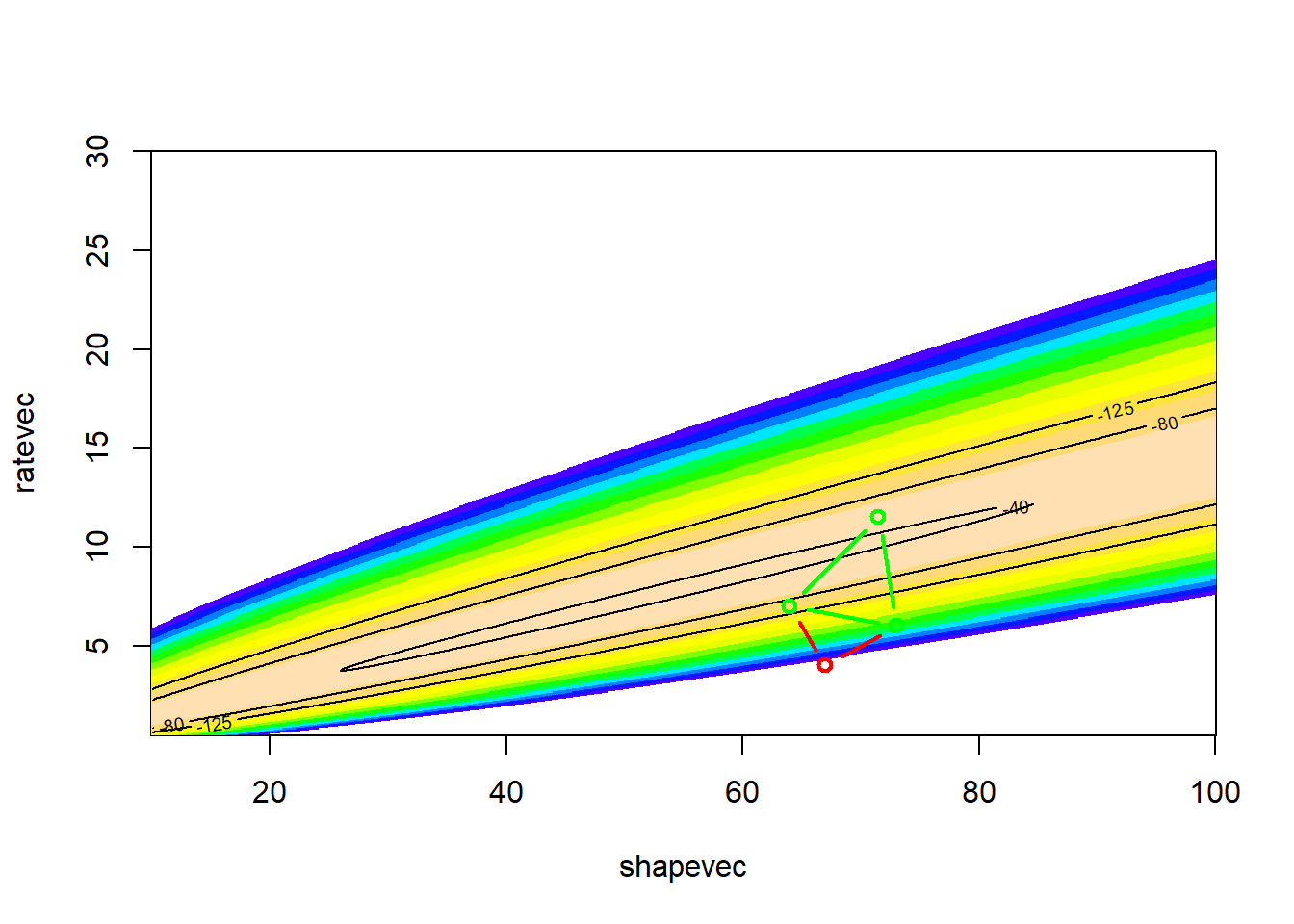And again! ############ # Make another move oldsimplex <- newsimplex newsimplex <- MoveTheSimplex(oldsimplex) ## Warning in dgamma(Myx$titer, shape = params["shape"], scale =
## params["scale"], : NaNs produced
image(x=shapevec,y=scalevec,z=surface2D,zlim=c(-1000,-30),col=topo.colors(12))
addSimplex(newsimplex)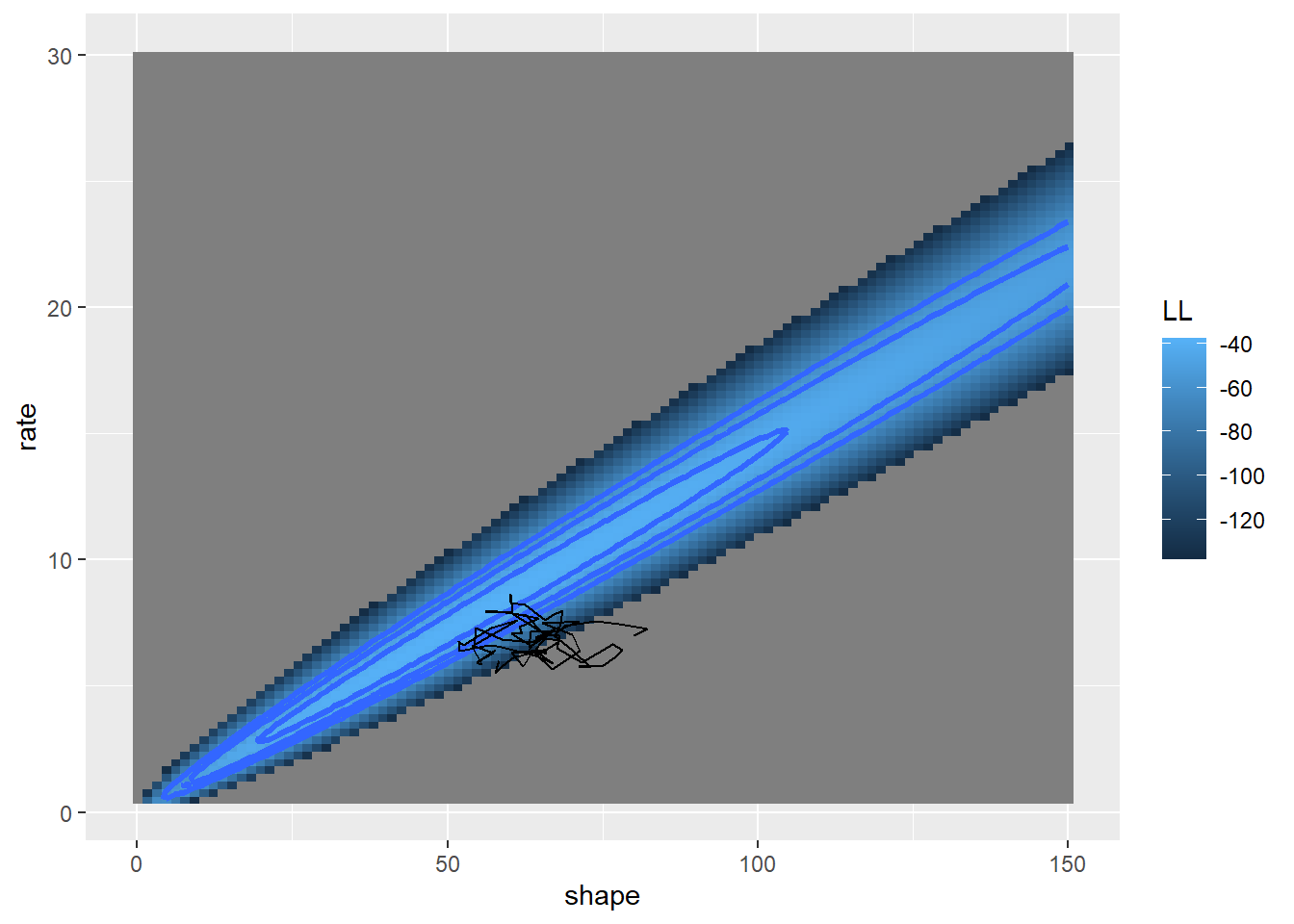Again:

############
# Make another move

oldsimplex <- newsimplex
newsimplex <- MoveTheSimplex(oldsimplex)
## Warning in dgamma(Myx$titer, shape = params["shape"], scale = ## params["scale"], : NaNs produced image(x=shapevec,y=scalevec,z=surface2D,zlim=c(-1000,-30),col=topo.colors(12)) contour(x=shapevec,y=scalevec,z=surface2D,levels=c(-30,-40,-80,-500),add=T) addSimplex(oldsimplex,col="red") addSimplex(newsimplex)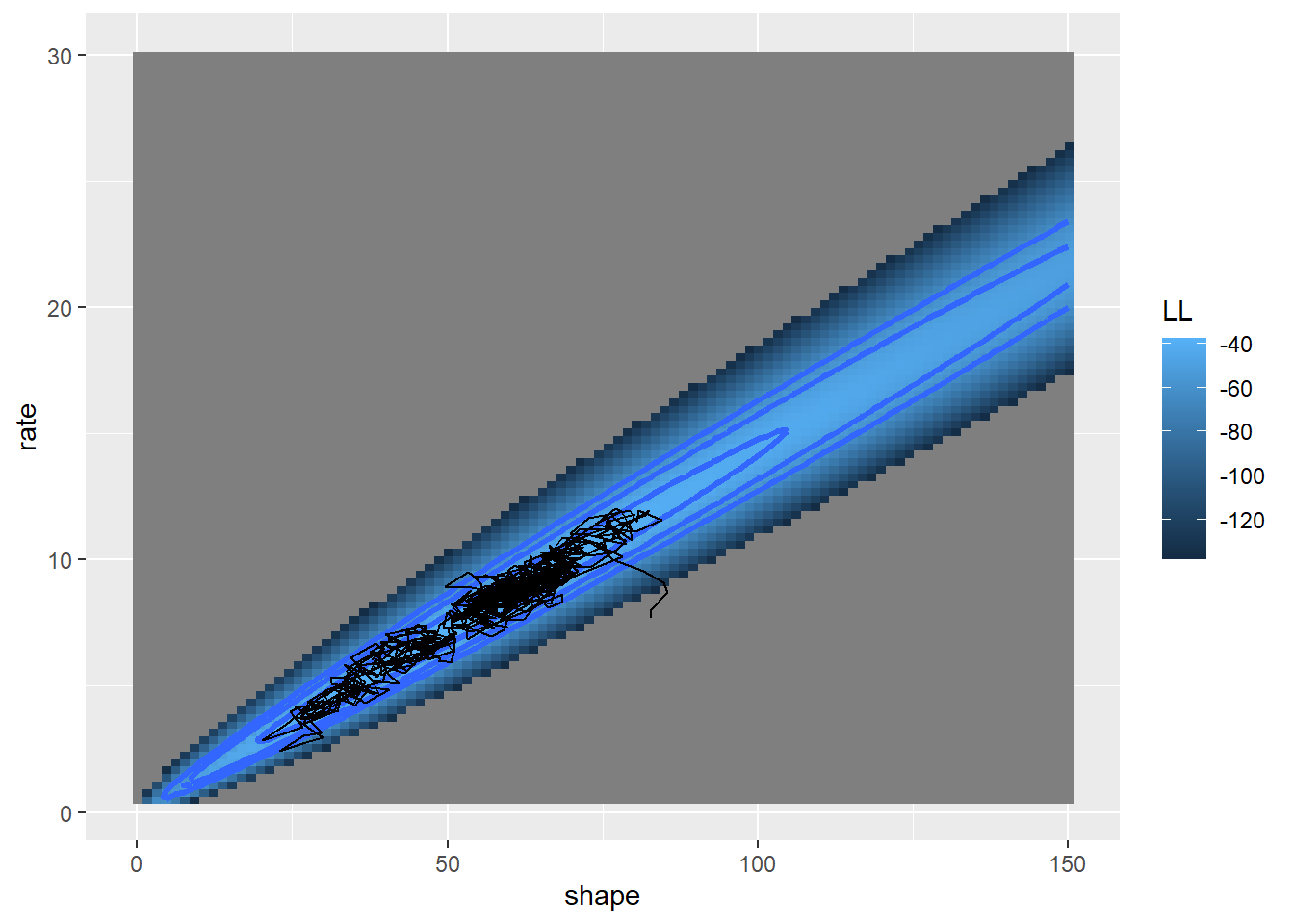And another few times: ############ # Make another few moves par(mfrow=c(2,2)) for(i in 1:4){ oldsimplex <- newsimplex newsimplex <- MoveTheSimplex(oldsimplex) image(x=shapevec,y=scalevec,z=surface2D,zlim=c(-1000,-30),col=topo.colors(12)) contour(x=shapevec,y=scalevec,z=surface2D,levels=c(-30,-40,-80,-500),add=T) addSimplex(oldsimplex,col="red") addSimplex(newsimplex) } ## Warning in dgamma(Myx$titer, shape = params["shape"], scale =
## params["scale"], : NaNs produced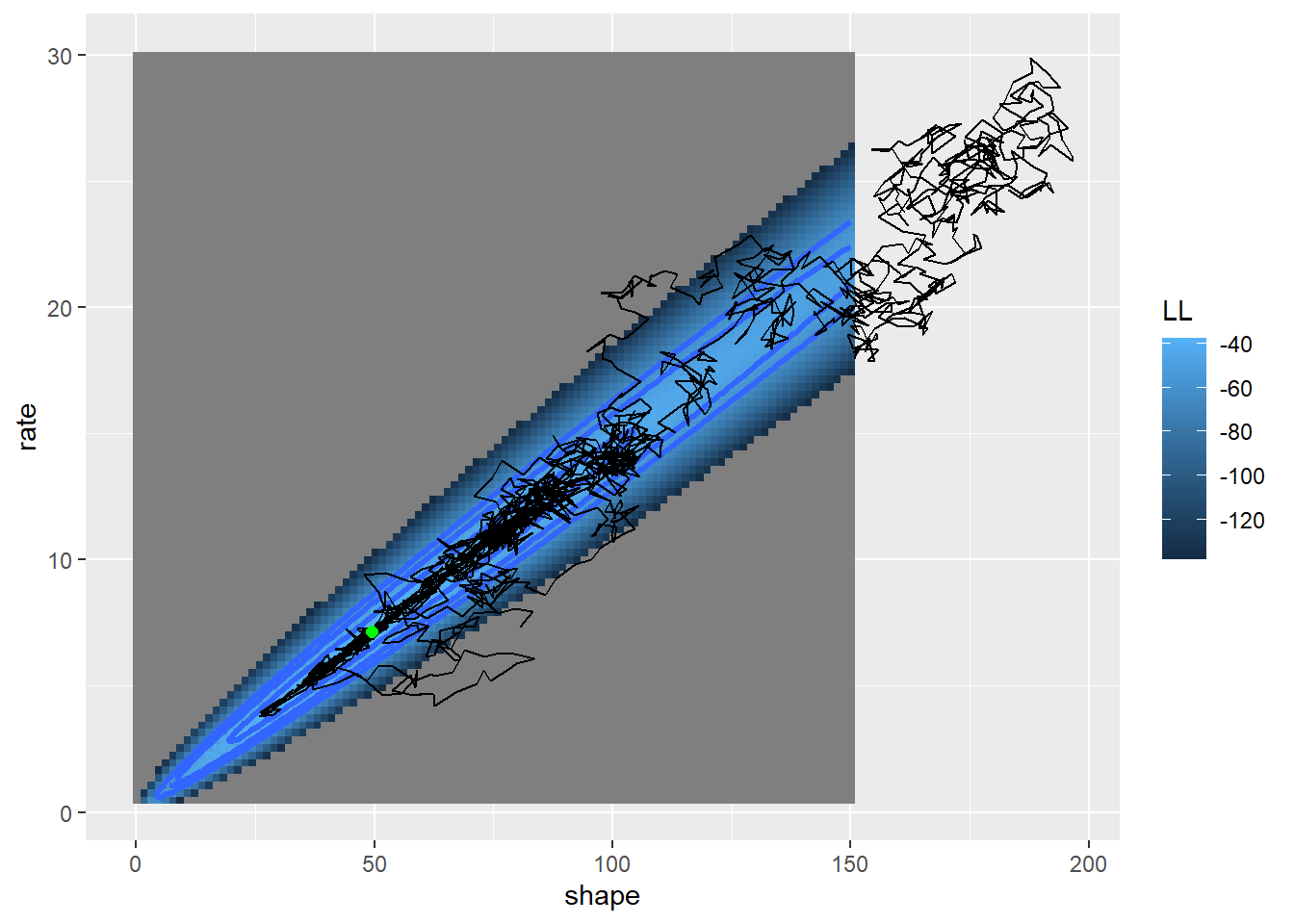Now we can build a function and use the algorithm for optimizing!

############
# Build a simplex optimization function!

SimplexMethod <- function(firstguess,tolerance=0.00001){
initsimplex <- list()
initsimplex[['vertex1']] <- firstguess + c(5,0.05)
initsimplex[['vertex2']] <- firstguess + c(-5,-0.05)
initsimplex[['vertex3']] <- firstguess + c(5,-0.05)
VertexLik <- SimplexLik(initsimplex)
oldbestlik <- VertexLik[which.max(VertexLik)]
deltalik <- 100
counter <- 0
while(counter<100){
newsimplex <- MoveTheSimplex(oldsimplex)
VertexLik <- SimplexLik(newsimplex)
bestlik <- VertexLik[which.max(VertexLik)]
deltalik <- bestlik-oldbestlik
oldsimplex <- newsimplex
oldbestlik <- bestlik
counter <- counter+1
}
mle <- list()
mle$estimate <- newsimplex[] mle$likelihood <- bestlik
mle$iterations <- counter return(mle) } SimplexMethod(firstguess = c(shape=39,scale=0.28)) ##$estimate
##     shape     scale
## 49.611453  0.139566
##
## $likelihood ## vertex2 ## -37.66714 ## ##$iterations
##  100

Okay, it’s close but doesn’t seem to be optimizing very well compared with the other methods. I probably didn’t implement it perfectly yet!

I like to call this the “amoeba” method of optimization??

In general, the simplex-based methods are less efficient than the derivative-based methods at finding the MLE- especially as you near the MLE.

## Derivative-free method 2: simulated annealing (SE).

Simulated annealing is one of my favorite optimization techniques. I think it serves as a good metaphor for problem-solving in general. When solving a problem, the first step is to think big, try to imagine whether we might be missing possible solutions. Then we settle (focus) on a general solution, learn more about how that solution applies to our problem, and ultimately get it done!

The temperature analogy is fun too! We start out “hot”- unfocused, frenzied, bouncing around - and we end up “cold” - crystal clear and focused on a solution!

### SE: A “global” optimization solution

Simulated annealing is called a “global” optimization solution because it can deal with false peaks and other strangenesses that can arise in optimization problems (e.g., maximizing likelihood). The price is in reduced efficiency!

#### Pseudocode for the Metropolis simulated annealing routine

Pick an initial starting point and evaluate the likelihood.

• Pick a new point at random near your old point and compute the (log) likelihood
• If the new value is better, accept it and start again
• If the new value is worse, then
- Pick a random number between zero and 1
- Accept the new (worse) value anyway if the random number is less than exp(change in log likelihood/k). Otherwise, go back to the previous value
• Periodically (e.g. every 100 iterations) lower the value of k to make it harder to accept bad moves. Eventually, the algorithm will “settle down” on a particular point in parameter space.

A simulated annealing method is available in the “optim” function in R (method = “SANN”)

### Example: Simulated annealing!

Let’s use the same familiar myxomatosis example!

################
# Simulated annealing!

startingvals <- c(shape=80,scale=0.15)
startinglik <- GammaLikelihoodFunction(startingvals)
startinglik
##  -313.6188
k = 100   # set the "temperature"

# function for making new guesses
newGuess <- function(oldguess=startingvals){
maxshapejump <- 5
maxscalejump <- 0.05
jump <- c(runif(1,-maxshapejump,maxshapejump),runif(1,-maxscalejump,maxscalejump))
newguess <- oldguess + jump
return(newguess)
}
# set a new "guess" near to the original guess

newGuess(oldguess=startingvals)     # each time is different- this is the first optimization procedure with randomness built in
##      shape      scale
## 84.7860456  0.1919917
newGuess(oldguess=startingvals)
##      shape      scale
## 76.0861265  0.1601342
newGuess(oldguess=startingvals)
##      shape      scale
## 77.1531882  0.1557441

Now let’s evaluate the difference in likelihood between the old and the new guess…

############
# evaluate the difference in likelihood between the new proposal and the old point

LikDif <- function(oldguess,newguess){
oldLik <- GammaLikelihoodFunction(oldguess)
newLik <- GammaLikelihoodFunction(newguess)
return(newLik-oldLik)
}

newguess <- newGuess(oldguess=startingvals)
loglikdif <- LikDif(oldguess=startingvals,newguess)
loglikdif
##  -101.854

Now let’s look at the Metropolis routine:

############
# run and visualize a Metropolis routine

k <- 100
oldguess <- startingvals
counter <- 0
guesses <- matrix(0,nrow=100,ncol=2)
colnames(guesses) <- names(startingvals)
while(counter<100){
newguess <- newGuess(oldguess)
loglikdif <- LikDif(oldguess,newguess)
if(loglikdif>0){
oldguess <- newguess
}else{
rand=runif(1)
if(rand <= exp(loglikdif/k)){
oldguess <- newguess   # accept even if worse!
}
}
counter <- counter + 1
guesses[counter,] <- oldguess
}

# visualize!

image(x=shapevec,y=scalevec,z=surface2D,zlim=c(-1000,-30),col=topo.colors(12))
lines(guesses,col="red")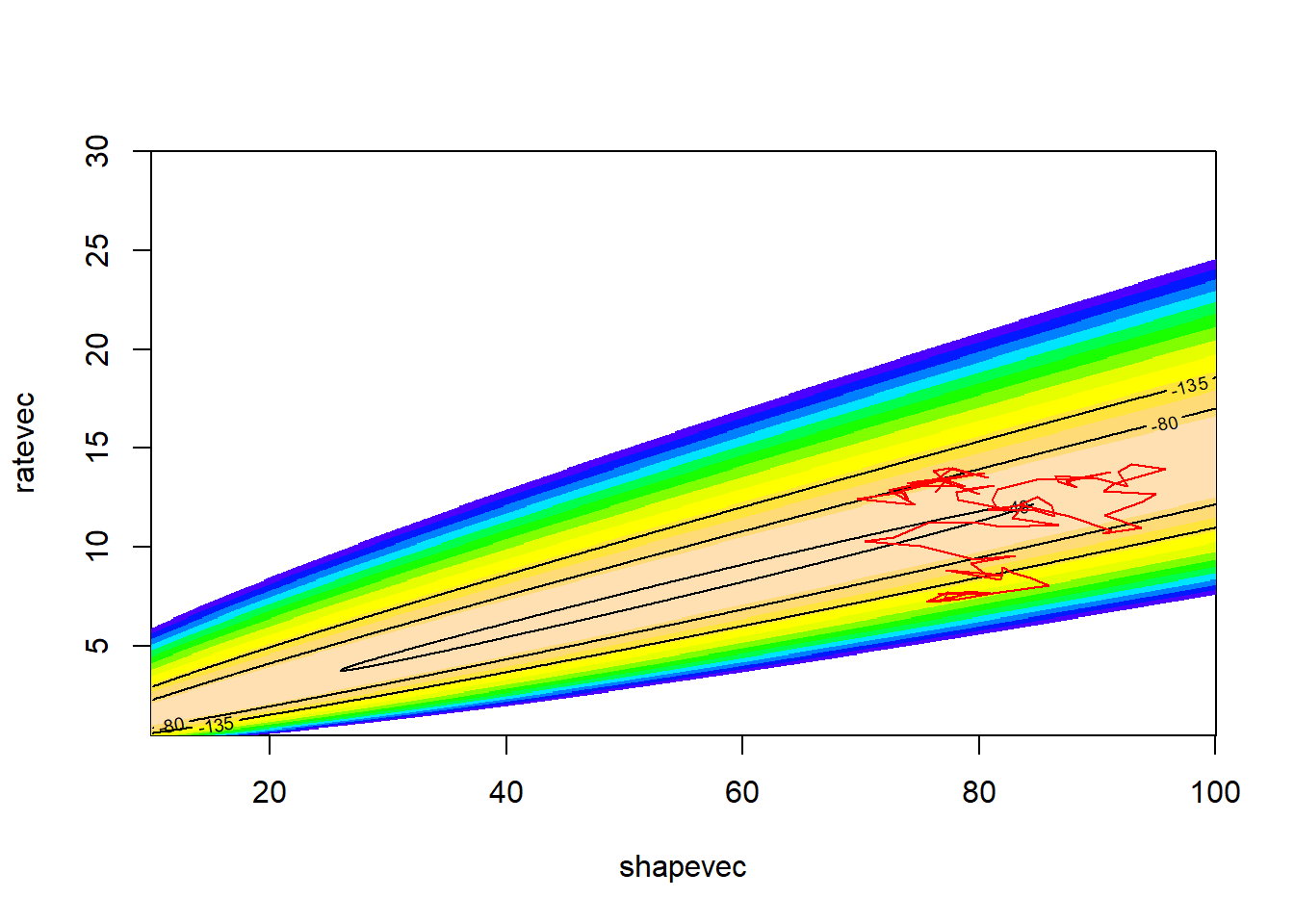Clearly this is the most inefficient, brute-force method we have seen so far (aside from the actual brute force method). And also quite clearly, in the context of this class, the best and most fun (and dangerous!).

NOTE: simulated annealing is way more efficient than the brute force method we saw earlier, especially with multiple dimensions!

Let’s run it for longer, and with a smaller value of k..

###########
# Run it for longer!

k <- 10
oldguess <- startingvals
counter <- 0
guesses <- matrix(0,nrow=1000,ncol=2)
colnames(guesses) <- names(startingvals)
while(counter<1000){
newguess <- newGuess(oldguess)
loglikdif <- LikDif(oldguess,newguess)
if(loglikdif>0){
oldguess <- newguess
}else{
rand=runif(1)
if(rand <= exp(loglikdif/k)){
oldguess <- newguess   # accept even if worse!
}
}
counter <- counter + 1
guesses[counter,] <- oldguess
}

# visualize!

image(x=shapevec,y=scalevec,z=surface2D,zlim=c(-1000,-30),col=topo.colors(12))
lines(guesses,col="red")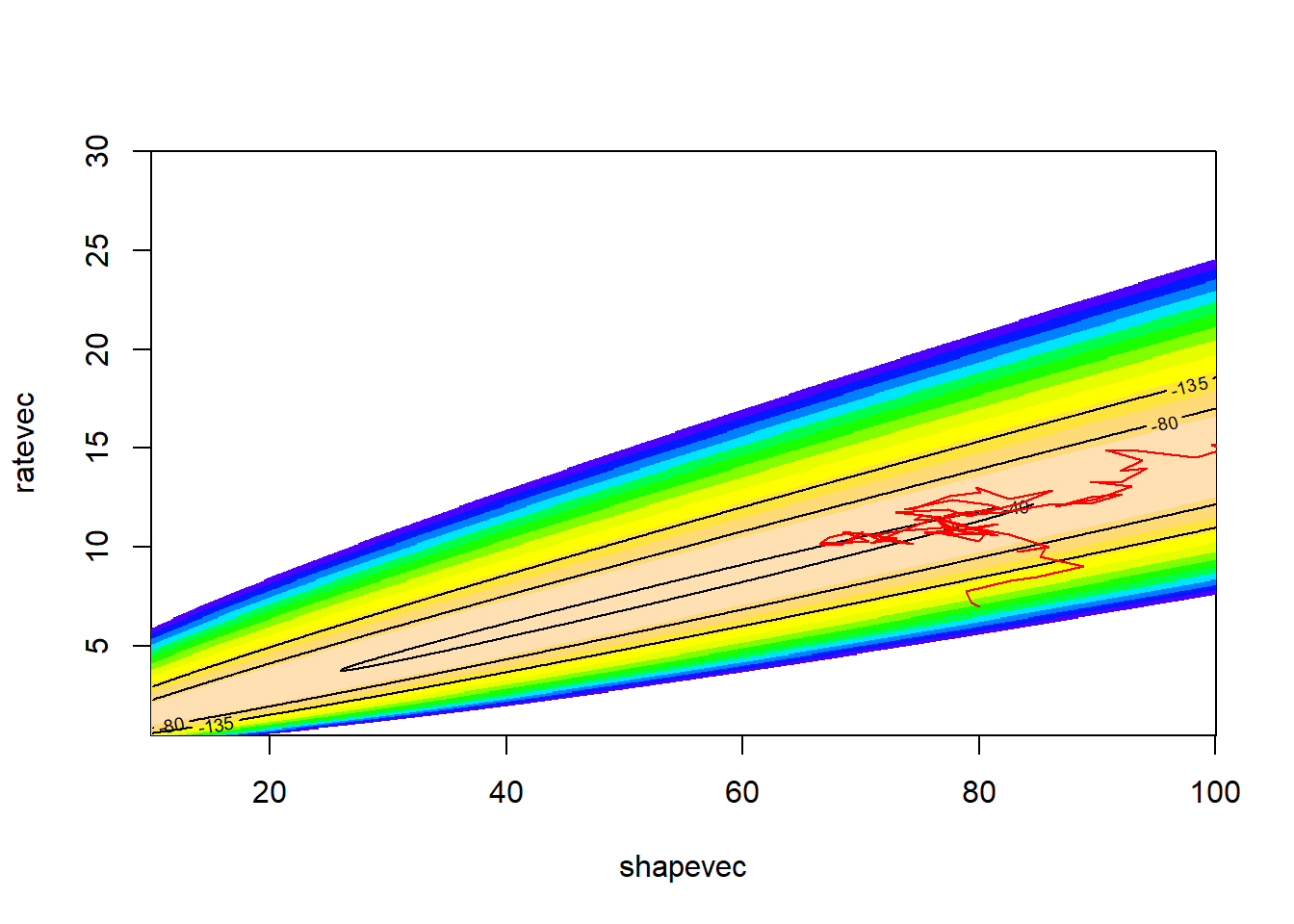This looks better! The search algorithm is finding the high-likelihood parts of parameter space pretty well!

Now let’s “cool” the temperature over time, let the algorithm settle down on a likelihood peak

#############
# cool the "temperature" over time and let the algorithm settle down

k <- 100
oldguess <- startingvals
counter <- 0
guesses <- matrix(0,nrow=10000,ncol=2)
colnames(guesses) <- names(startingvals)
MLE <- list(vals=startingvals,lik=GammaLikelihoodFunction(startingvals),step=0)
while(counter<10000){
newguess <- newGuess(oldguess)
loglikdif <- LikDif(oldguess,newguess)
if(loglikdif>0){
oldguess <- newguess
}else{
rand=runif(1)
if(rand <= exp(loglikdif/k)){
oldguess <- newguess   # accept even if worse!
}
}
counter <- counter + 1
if(counter%%100==0) k <- k*0.8
guesses[counter,] <- oldguess
thislik <- GammaLikelihoodFunction(oldguess)
if(thislik>MLE$lik) MLE <- list(vals=oldguess,lik=GammaLikelihoodFunction(oldguess),step=counter) } # visualize! image(x=shapevec,y=scalevec,z=surface2D,zlim=c(-1000,-30),col=topo.colors(12)) contour(x=shapevec,y=scalevec,z=surface2D,levels=c(-30,-40,-80,-500),add=T) lines(guesses,col="red") points(MLE$vals,MLE$vals,col="green",pch=20,cex=3)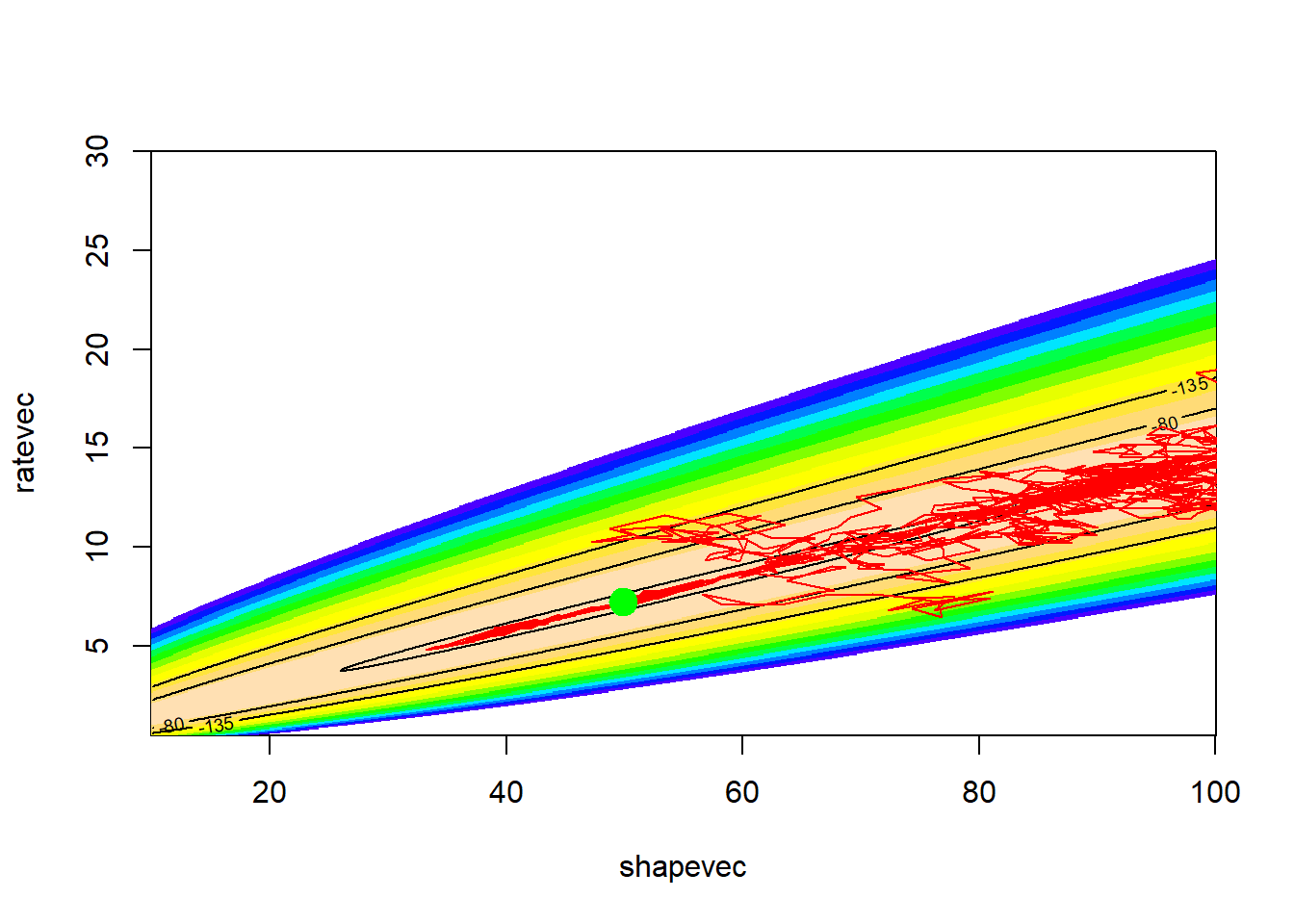MLE ##$vals
##      shape      scale
## 49.7993557  0.1389658
##
## $lik ##  -37.66742 ## ##$step
##  9200

As you can see, the simulated annealing method did pretty well. However, we needed thousands of iterations to do what other methods just take a few iterations to do. But, we might feel better that we have explored parameter space more thoroughly and avoided the potential problem of false peaks (although there’s no guarantee that the simulated annealing method will find the true MLE).

## Other methods

As you can see, there are many ways to optimize- and the optimal optimization routine is not always obvious!

You can probably use some creative thinking and imagine your own optimization algorithm… For example, some have suggested combining the simplex method with the simulated annealing method! Optimization is an art!!

## What about the confidence interval??

As you can see in the previous examples, most of the optimization techniques we have looked at do not explore parameter space enough to discern the shape of the likelihood surface around the maximum likelihood estimate. Therefore, we do not have the information we need to compute the confidence intervals around our parameter estimates. And what good is a point estimate without a corresponding estimate of uncertainty??

There are several techniques that are widely used to estimate and describe parameter uncertainty:

1. Brute force (expose the entire likelihood surface!) [okay, this one isn’t actually used very often]
2. Profile likelihood (the most accurate way!)
3. Evaluate curvature at the MLE and use that to estimate sampling error (somewhat inexact but efficient- but generally performs pretty well, and is the default for many MLE routines!)

–go to next lecture–